Anzeige

# Introduction to communication system lecture1

27. May 2016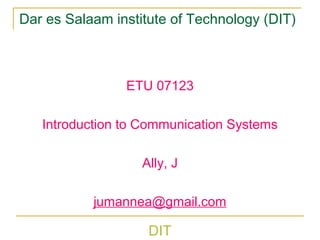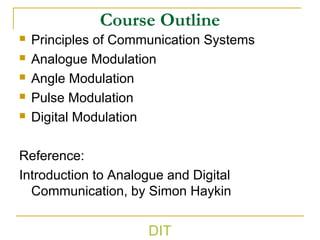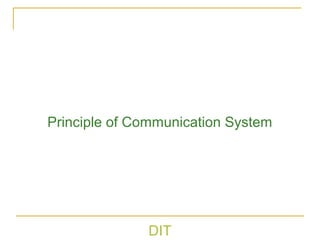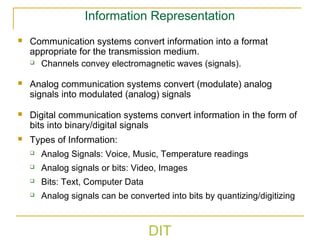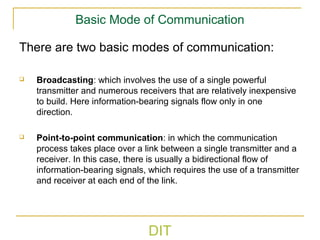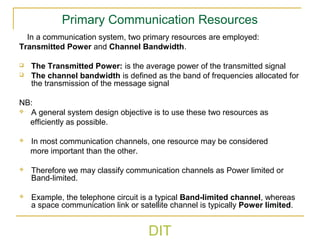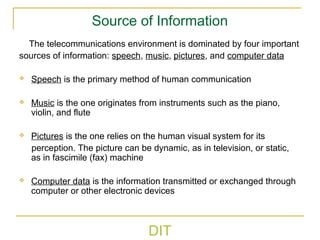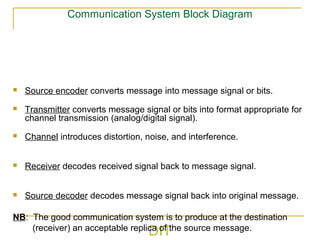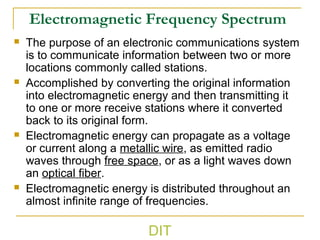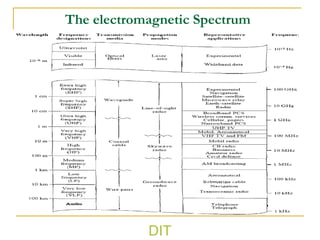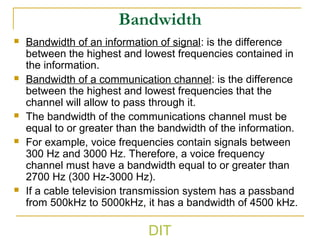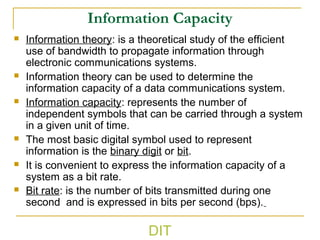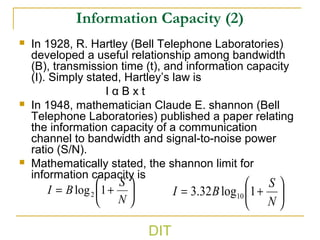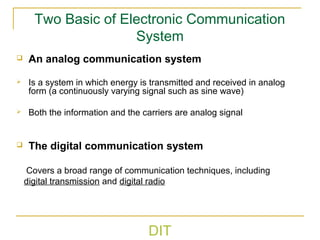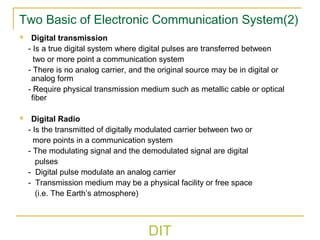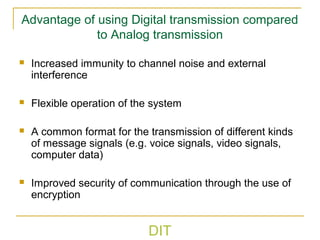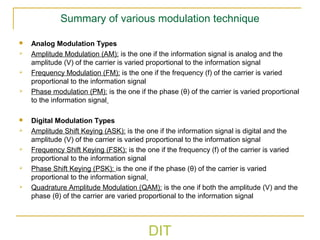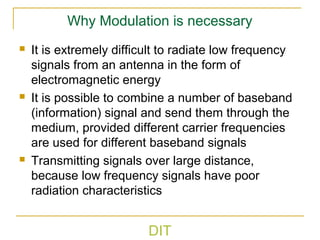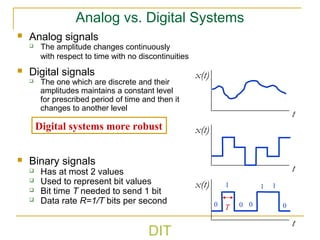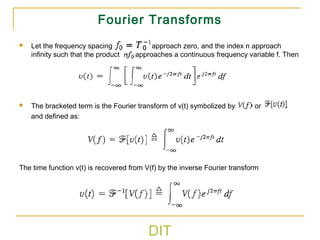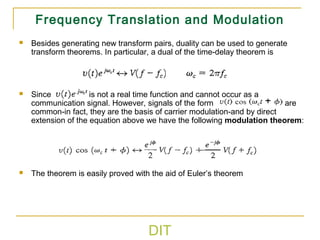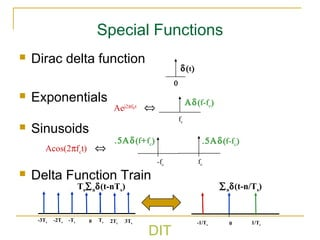1 von 24
Anzeige

### Introduction to communication system lecture1

1. DIT Dar es Salaam institute of Technology (DIT) ETU 07123 Introduction to Communication Systems Ally, J jumannea@gmail.com
2. Course Outline  Principles of Communication Systems  Analogue Modulation  Angle Modulation  Pulse Modulation  Digital Modulation Reference: Introduction to Analogue and Digital Communication, by Simon Haykin DIT
3. DIT Principle of Communication System
4. DIT Information Representation  Communication systems convert information into a format appropriate for the transmission medium.  Channels convey electromagnetic waves (signals).  Analog communication systems convert (modulate) analog signals into modulated (analog) signals  Digital communication systems convert information in the form of bits into binary/digital signals  Types of Information:  Analog Signals: Voice, Music, Temperature readings  Analog signals or bits: Video, Images  Bits: Text, Computer Data  Analog signals can be converted into bits by quantizing/digitizing
5. DIT Basic Mode of Communication There are two basic modes of communication:  Broadcasting: which involves the use of a single powerful transmitter and numerous receivers that are relatively inexpensive to build. Here information-bearing signals flow only in one direction.  Point-to-point communication: in which the communication process takes place over a link between a single transmitter and a receiver. In this case, there is usually a bidirectional flow of information-bearing signals, which requires the use of a transmitter and receiver at each end of the link.
6. DIT Primary Communication Resources In a communication system, two primary resources are employed: Transmitted Power and Channel Bandwidth.  The Transmitted Power: is the average power of the transmitted signal  The channel bandwidth is defined as the band of frequencies allocated for the transmission of the message signal NB:  A general system design objective is to use these two resources as efficiently as possible.  In most communication channels, one resource may be considered more important than the other.  Therefore we may classify communication channels as Power limited or Band-limited.  Example, the telephone circuit is a typical Band-limited channel, whereas a space communication link or satellite channel is typically Power limited.
7. DIT Source of Information The telecommunications environment is dominated by four important sources of information: speech, music, pictures, and computer data  Speech is the primary method of human communication  Music is the one originates from instruments such as the piano, violin, and flute  Pictures is the one relies on the human visual system for its perception. The picture can be dynamic, as in television, or static, as in fascimile (fax) machine  Computer data is the information transmitted or exchanged through computer or other electronic devices
8. DIT Communication System Block Diagram  Source encoder converts message into message signal or bits.  Transmitter converts message signal or bits into format appropriate for channel transmission (analog/digital signal).  Channel introduces distortion, noise, and interference.  Receiver decodes received signal back to message signal.  Source decoder decodes message signal back into original message. NB: The good communication system is to produce at the destination (receiver) an acceptable replica of the source message.
9. DIT Modulation and Demodulation  Modulation  Is the process of changing ore or more properties such as amplitude, frequency, and phase of the analog carrier in proportion with the information signal  Performed in a transmitter by a circuit called a modulator  Demodulation  Is the reverse process of modulation and converts the modulated carrier back to the original information  Performed in a receiver by a circuit called a demodulator
10. DIT Electromagnetic Frequency Spectrum  The purpose of an electronic communications system is to communicate information between two or more locations commonly called stations.  Accomplished by converting the original information into electromagnetic energy and then transmitting it to one or more receive stations where it converted back to its original form.  Electromagnetic energy can propagate as a voltage or current along a metallic wire, as emitted radio waves through free space, or as a light waves down an optical fiber.  Electromagnetic energy is distributed throughout an almost infinite range of frequencies.
11. DIT The electromagnetic Spectrum
12. DIT Bandwidth  Bandwidth of an information of signal: is the difference between the highest and lowest frequencies contained in the information.  Bandwidth of a communication channel: is the difference between the highest and lowest frequencies that the channel will allow to pass through it.  The bandwidth of the communications channel must be equal to or greater than the bandwidth of the information.  For example, voice frequencies contain signals between 300 Hz and 3000 Hz. Therefore, a voice frequency channel must have a bandwidth equal to or greater than 2700 Hz (300 Hz-3000 Hz).  If a cable television transmission system has a passband from 500kHz to 5000kHz, it has a bandwidth of 4500 kHz.
13. DIT Information Capacity  Information theory: is a theoretical study of the efficient use of bandwidth to propagate information through electronic communications systems.  Information theory can be used to determine the information capacity of a data communications system.  Information capacity: represents the number of independent symbols that can be carried through a system in a given unit of time.  The most basic digital symbol used to represent information is the binary digit or bit.  It is convenient to express the information capacity of a system as a bit rate.  Bit rate: is the number of bits transmitted during one second and is expressed in bits per second (bps).
14. DIT Information Capacity (2)  In 1928, R. Hartley (Bell Telephone Laboratories) developed a useful relationship among bandwidth (B), transmission time (t), and information capacity (I). Simply stated, Hartley’s law is I α B x t  In 1948, mathematician Claude E. shannon (Bell Telephone Laboratories) published a paper relating the information capacity of a communication channel to bandwidth and signal-to-noise power ratio (S/N).  Mathematically stated, the shannon limit for information capacity is       += N S BI 1log2       += N S BI 1log32.3 10
15. DIT Two Basic of Electronic Communication System  An analog communication system  Is a system in which energy is transmitted and received in analog form (a continuously varying signal such as sine wave)  Both the information and the carriers are analog signal  The digital communication system Covers a broad range of communication techniques, including digital transmission and digital radio
16. DIT Two Basic of Electronic Communication System(2)  Digital transmission - Is a true digital system where digital pulses are transferred between two or more point a communication system - There is no analog carrier, and the original source may be in digital or analog form - Require physical transmission medium such as metallic cable or optical fiber  Digital Radio - Is the transmitted of digitally modulated carrier between two or more points in a communication system - The modulating signal and the demodulated signal are digital pulses - Digital pulse modulate an analog carrier - Transmission medium may be a physical facility or free space (i.e. The Earth’s atmosphere)
17. DIT Advantage of using Digital transmission compared to Analog transmission  Increased immunity to channel noise and external interference  Flexible operation of the system  A common format for the transmission of different kinds of message signals (e.g. voice signals, video signals, computer data)  Improved security of communication through the use of encryption
18. DIT Summary of various modulation technique  Analog Modulation Types  Amplitude Modulation (AM): is the one if the information signal is analog and the amplitude (V) of the carrier is varied proportional to the information signal  Frequency Modulation (FM): is the one if the frequency (f) of the carrier is varied proportional to the information signal  Phase modulation (PM): is the one if the phase (θ) of the carrier is varied proportional to the information signal  Digital Modulation Types  Amplitude Shift Keying (ASK): is the one if the information signal is digital and the amplitude (V) of the carrier is varied proportional to the information signal  Frequency Shift Keying (FSK): is the one if the frequency (f) of the carrier is varied proportional to the information signal  Phase Shift Keying (PSK): is the one if the phase (θ) of the carrier is varied proportional to the information signal  Quadrature Amplitude Modulation (QAM): is the one if both the amplitude (V) and the phase (θ) of the carrier are varied proportional to the information signal
19. DIT Why Modulation is necessary  It is extremely difficult to radiate low frequency signals from an antenna in the form of electromagnetic energy  It is possible to combine a number of baseband (information) signal and send them through the medium, provided different carrier frequencies are used for different baseband signals  Transmitting signals over large distance, because low frequency signals have poor radiation characteristics
20. DIT Analog vs. Digital Systems  Analog signals  The amplitude changes continuously with respect to time with no discontinuities  Digital signals  The one which are discrete and their amplitudes maintains a constant level for prescribed period of time and then it changes to another level  Binary signals  Has at most 2 values  Used to represent bit values  Bit time T needed to send 1 bit  Data rate R=1/T bits per second t x(t) t x(t) t x(t) 1 0 0 0 1 1 0T Digital systems more robust
21. DIT Fourier Transforms  Let the frequency spacing approach zero, and the index n approach infinity such that the product approaches a continuous frequency variable f. Then  The bracketed term is the Fourier transform of v(t) symbolized by or and defined as: The time function v(t) is recovered from V(f) by the inverse Fourier transform
22. DIT Frequency Translation and Modulation  Besides generating new transform pairs, duality can be used to generate transform theorems. In particular, a dual of the time-delay theorem is  Since is not a real time function and cannot occur as a communication signal. However, signals of the form are common-in fact, they are the basis of carrier modulation-and by direct extension of the equation above we have the following modulation theorem:  The theorem is easily proved with the aid of Euler’s theorem
23. DIT Special Functions  Dirac delta function  Exponentials  Sinusoids  Delta Function Train δ(t) 0 fc Αδ(f-fc) Aej2πfct ⇔ Acos(2πfct) ⇔ fc .5Αδ(f-fc) -fc .5Αδ(f+fc) Ts∑nδ(t-nTs) 0 ∑nδ(t-n/Ts) 0 Ts 2Ts 3Ts -Ts-2Ts-3Ts 1/Ts-1/Ts
24. DIT

### Hinweis der Redaktion

1. Special Functions • The dirac delta function is a key function in Fourier analysis, as it described the impulse response of a filter. • An exponential in time becomes a delta function in the frequency domain at the frequency of the exponential. • Sinusoids become two delta functions at the positive and negative frequencies of the sinusoid. • A delta function train in the time domain becomes a delta function train in the frequency domain.
Anzeige